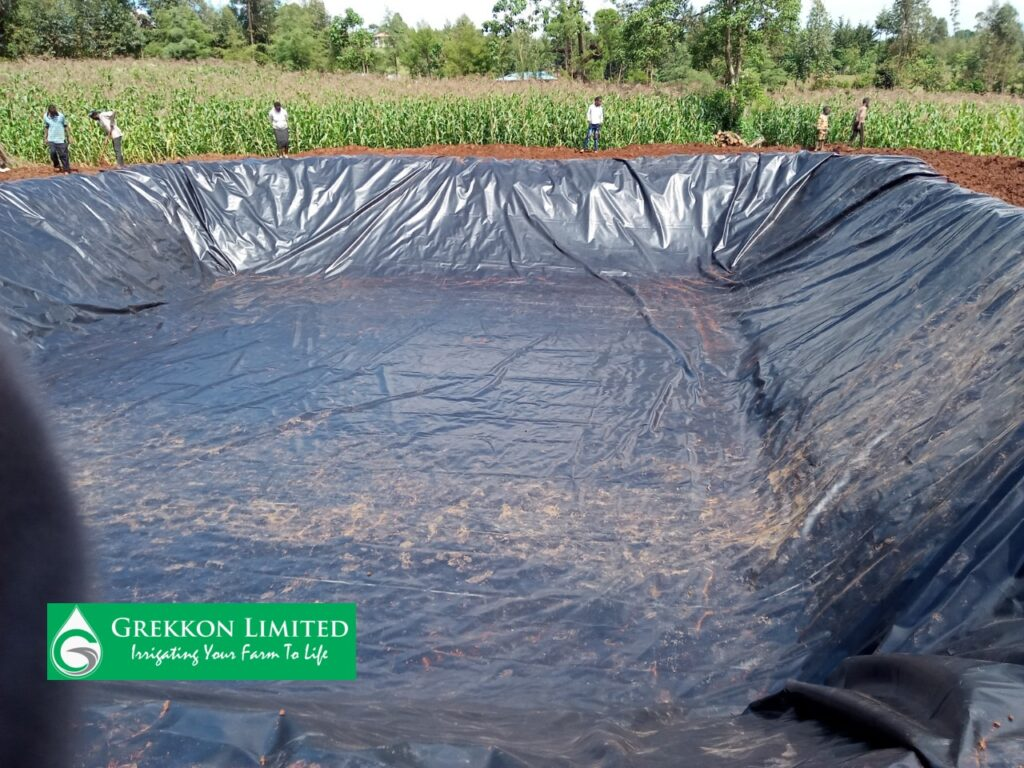Welcome To Grekkon Eldoret.

# Damliner Calculator

Damliner calculator formular is a simple mathematical formula used by farmers, technicians and engineers to determine the size of dam liner required for dam lining. Damliner calculator formular takes into consideration the length, width and the depth for the reservoir to get the final size dam liner required in m sq. The dam liner calculator for rectangular and cylindrical reservoirs is almost the same. These methods explained below will ease all the problems of material under estimation or over estimation during dam liner installations.

#### How to Calculate Damliner required for deep rectangular reservoirs/ponds

To determine the damliner size required, use the pond dam liner calculator formula:

1. Length (Longer side) add overall length plus twice the maximum depth plus allowance of a meter or more on either side for overlap
2. Width: (Short side) add overall length plus twice the maximum depth plus allowance of a meter or more on either side for overlap

For example. Assuming you have a pond measuring 10m long by 4m wide and 2.5m deep. Dam liner required =

L= L+2*D+(1+1)

W= L+2*D+(1+1)

So,  L= 10+(2*2.5)+2

= 10+5+2

L =17m

So, W= 4+(2*2.5)+2

= 4+5+2

W=11m

So pond dam liner required= 17m x 11m.

NB: 2m is the allowance for overlap to ensure the dam liner is properly secured on the edges.

#### How to Calculate Dam liner Required for Cylindrical/Circular Reservoirs

For cylindrical tanks, just add the diameter of the tank plus twice the height plus an allowance on either side for overlap.

For Example

Tank Diameter= 10m

Tank Height= 2m

Allowance for overlap for one side= 0.5m

Dam liner required

L= 10m + (2+2)+ (0.5+0.5)

=10+4+1

=15m

Since it’s a circular tank, the length equals the width, so width = 15m.

So Dam liner required = 15m by 15m = 225m2

Please note that circular reservoirs use more dam liners and carry less water than rectangular/square structures for the same liner size. So always strive to have rectangular or square reservoirs.

#### Pond liner Calculator for Reservoirs with Slanted Heights.

For dam liners with slanted heights, simply use the pond liner calculator formular:

• Length= Length of the base (B1) plus twice the slanting height plus an allowance on either side
• Width = Width of the base plus twice the slanting height plus an allowance on either side.

The photo below shows an example of such reservoirs.A reservoir with slanted slope

For example. Assuming you have a pond whose base measurements are: Base length (B1) = 13m, Base width (B2) = 7m and slanting height (H) as 3m;

L= B1+2*H+(1+1)

W= B2+2*H+(1+1)

So,  L= 13+(2*3)+2

= 13+6+2

L =21m

So, W= B2+(2*3)+2

= 7+6+2

W=15m

So dam liner required= 21m x 15m.

= 315m sq

NB:

• 2m is the allowance for overlap to ensure the dam liner is properly secured on the edges. Note that the allowance is not always fixed, you may encounter reservoirs that need more or less dam liners.
• Also note that for reservoirs with slanted height, the upper length and width have no use when calculating dam liner required, so you will have to ignore them.

### Related posts

1.#### Khalil Dukuly

Many thanks for your quick response. I wanted to make the depth 1.5 meters to reduce the risk of accidents on the farm. I also did not understand what you meant by slanting the sides. I appreciate your valuable assistance and support in this matter. Is this calculation feasible? 43 meters in Length, 8 meters in width, 1.5 meters in depth and 2 meters overlay.

2.#### Khalil Dukuly

Hello, many thanks for this useful information. I am from Liberia and there are no company providing such information about dam reservoirs. I am wondering if you could calculate my pond size. I have purchased liners with the dimensions of 15 meters in width and 50 meters in length.

Many thanks and best regards, Khalil

•